How Cheenta works to ensure student success?
Explore the Back-Story

# TIFR 2014 Problem 28 Solution - Continuous Functions from Discrete SpaceTIFR 2014 Problem 28 Solution is a part of TIFR entrance preparation series. The Tata Institute of Fundamental Research is India's premier institution for advanced research in Mathematics. The Institute runs a graduate programme leading to the award of Ph.D., Integrated M.Sc.-Ph.D. as well as M.Sc. degree in certain subjects.
The image is a front cover of a book named Introduction to Real Analysis by R.G. Bartle, D.R. Sherbert. This book is very useful for the preparation of TIFR Entrance.

Also Visit: College Mathematics Program of Cheenta

## Problem:

Let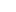be a topological space such that every function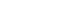is continuous. Then

A.has the discrete topology.

B.has the indiscrete topology.

C.is compact.

D.is not connected.

Discussion:

We know that if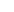is a discrete space then any function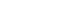is continuous.

Option A asks whether the converse to this is true in the case that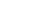.

To prove/disprove whetherhas the discrete topology or not it is enough to prove whether every singleton set is open or not.

If we can show that for every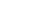there exists a function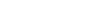such that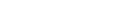then we are done. Because we are given that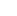if exists must be continuous, and since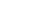is open in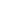we will have the inverse image of it open in, so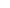will be open in.

Now, this target is easy to handle. We define for each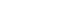and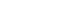for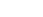.

Thissatisfies our desired property. Sois discrete.

Taking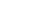(for example) shows thatdoes not need to be indiscrete nor does it have to be compact.

Takingshows thatmay be connected. Of course ifhas cardinality more than 1, it is not connected.

## Helpdesk

• What is this topic: Real Analysis
• What are some of the associated concept: Continuity, Discrete Space
• Book Suggestions: Introduction to Real Analysis by R.G. Bartle, D.R. SherbertTIFR 2014 Problem 28 Solution is a part of TIFR entrance preparation series. The Tata Institute of Fundamental Research is India's premier institution for advanced research in Mathematics. The Institute runs a graduate programme leading to the award of Ph.D., Integrated M.Sc.-Ph.D. as well as M.Sc. degree in certain subjects.
The image is a front cover of a book named Introduction to Real Analysis by R.G. Bartle, D.R. Sherbert. This book is very useful for the preparation of TIFR Entrance.

Also Visit: College Mathematics Program of Cheenta

## Problem:

Letbe a topological space such that every functionis continuous. Then

A.has the discrete topology.

B.has the indiscrete topology.

C.is compact.

D.is not connected.

Discussion:

We know that ifis a discrete space then any functionis continuous.

Option A asks whether the converse to this is true in the case that.

To prove/disprove whetherhas the discrete topology or not it is enough to prove whether every singleton set is open or not.

If we can show that for everythere exists a functionsuch thatthen we are done. Because we are given thatif exists must be continuous, and sinceis open inwe will have the inverse image of it open in, sowill be open in.

Now, this target is easy to handle. We define for eachandfor.

Thissatisfies our desired property. Sois discrete.

Taking(for example) shows thatdoes not need to be indiscrete nor does it have to be compact.

Takingshows thatmay be connected. Of course ifhas cardinality more than 1, it is not connected.

## Helpdesk

• What is this topic: Real Analysis
• What are some of the associated concept: Continuity, Discrete Space
• Book Suggestions: Introduction to Real Analysis by R.G. Bartle, D.R. Sherbert

This site uses Akismet to reduce spam. Learn how your comment data is processed.

### Knowledge Partner